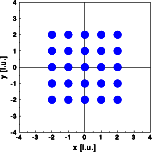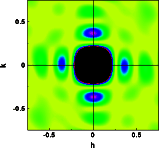Interactive Tutorial about Diffraction Convolution II: Square boxConvolution Convolution theorem Square box Rectangular box Circular box Box at 45 degrees Goto Contents This example illustrates the effect of the convolution theorem. The infinite crystal is multiplied by a square box that is one inside and zero outside. This procedure creates a finite crystal of 5 by 5 unit cells (left image). The Fourier transform creates a reciprocal lattice that is extending ad infinitum. Each Bragg reflections is convoluted by the Fourier transform of the box function (right image). The Bragg reflections are overexposed in the given pictures. Click on the pictures to get a full screen version.The Fourier transform of a real space product infinite lattice . box function gives the convolution of the Fourier transforms of the terms in the product F(infinite lattice) * F(box function) = infinite reciprocal lattice * F(boxfunction) To prove this statement lets simulate the box function and calculate its Fourier transform. (As additional exercise, the students may be asked to analytically calculate the Fourier transform of a box function.) The box is simulated by filling the shape at random with a large number (800) of atoms (left image). The resulting electron density distribution fills the box fairly equally. Next The Fourier transform is calculated in a small section of reciprocal space near the origin. The calculated intensity distribution corresponds to the distribution of the subsidiary maxima observed in the Fourier transform above.The deviations from square symmetry are due to the limited statistics provided by the 800 atoms within the square box. © Th. Proffen and R.B. Neder, 2003